# Mathematics on the Web: The EzMath notation

Dave Raggett <dsr@w3.org>,
Davy Batsalle <batsalle@email.enst.fr>
27th November 1997

### Abstract

The Web lacks an effective means for embedding mathematical expressions in Web pages. Hitherto, authors have been forced to use bitmapped images which are slow to load, complex to produce, and look bad when printed.

EzMath provides an easy to learn notation for embedding mathematical expressions in Web pages. The notation is inspired by how expressions are spoken aloud, together with a few abbreviations for conciseness.

EzMath covers a widely used subset of mathematics and can be used to create MathML content tags conforming to the W3C mathematical markup language. EzMath focusses on the meaning of mathematical notation rather than just how it looks on paper (or screen).

EzMath is available as freeware from http://www.w3.org/People/Raggett/EzMath/

## 1. Computer Typesetting of Mathematics

This section describes some earlier work on using computers to typeset mathematical expressions. In each case we use the same example:

  EzMath:
limit as x tends to a of function f(x)


One of the first well known computer packages for typesetting mathematical formulae is eqn, developed by Bell Labs for use with the Unix typesetting system troff. Eqn uses a regular grammar that is surprisingly readable and helped to inspire the approach taken by EzMath. A comparison of EzMath and Eqn is given in section 3.4.

  Eqn:
lim from {x -> a} f(x)


Eqn was eclipsed by Donald Knuth's TEX. This offered much greater control over typesetting details and has become the most popular method for electronic typesetting of mathematics. TEX is a lot more cryptic, making heavy use of macros. Leslie Lampson's LaTEX is a common macro package for TEX. The American Mathematical Society has developed its own macro package and guidelines for using TEX called AMS-TEX.

  TEX:
\lim_{x \rightarrow a} f(x)


The SGML community came up with their own solutions, notably the ISO 12083 standard which grew out of earlier work on the AAP DTD.

  AAP Math:
<lim><op><rf>lim</rf></op><ll>x → a</ll><opd>f(x)</opd></lim>

ISO 12083:
<subform><roman>lim</roman></subform><bottom>x → a</bottom> f(x)


At the time of writing there is no direct way to include mathematical expressions in Web pages. As a result people resort to rendering expressions using other tools, such as Word or LaTEX and converting them into bitmapped images in the GIF format.

This has a number of serious drawbacks: It makes pages slow to download. It means you have to manage a number of GIF files as well as the HTML page they belong to. The pages look terrible when printed out (the high resolution of printed pages exacerbates the limitations of the bitmapped images). The font size for expressions is fixed and doesn't match the end-user's font preferences. There is no effective answer for people who can't see the images. The expressions can't be moved to a mathematical manipulation package.

## 2. Idiosyncrasies of mathematical notations

Conventional mathematical notations rely on a shared understanding by the mathematicians who write and read these notations. The notations are generally incomplete and ambiguous. The same symbols tend to be reused for different functions, especially in different fields of mathematics.

• In calculus f' stands for the first derivative of f  but can mean any other entity different from f in other fields.

• Is i an integer variable, the index of a matrix or the square root of -1? What about j ?

• What is the function of 2 in SU2,  log2 x   x2   x2   or as indices on a tensor?

I grateful acknowledge the use here of examples taken from .

Both TEX and ISO 12083 focus on the visual presentation of mathematics rather than its meaning. For instance, in TEX you would represent the second derivative of f with respect to x as:

{{d^2f} \over {dx^2}}


This is fine for people reading the visual rendering of the TEX markup, but causes difficulties for people with visual disabilities using a speech synthesiser, or for software that wants to manipulate the mathematical expressions. The problems stem from having to guess the intended meaning.

This is critical to the Web, which aims to make information accessible to all. A satisfactory solution for representing mathematical expressions for the Web must be able to be effectively rendered to a wide variety of media, including bitmapped displays, fixed character grid displays, Braille, paper and speech synthesisers.

The Web encourages the development of active documents. It should be possible to paste expressions into tools for mathematical manipulation, such as Mathematica and Maple. Web pages may also offer an ability to graph expressions or otherwise manipulate them in place.

## 3. Introduction to EzMath

EzMath is the outcome of work at MIT and HP Labs aimed at providing an easy to learn notation using plain text that would make sense by itself as well being straightforward to render to the screen or to a speech synthesiser. It is inspired by the way you would speak an expression if you were asked to read it over the phone.

The scope of EzMath is broadly speaking designed to meet the needs for most of the formulas used from kindergarten to the end of high school in the US. Further work is planned to extend the coverage to meet the needs of 1st and 2nd year undergraduates. For a discussion of the relationship between EzMath and the W3C MathML notation, see section 3.3

• Arithmetic, Algebra and Relations
• Calculus
• Set Theory
• Sequences and Series
• Trigonometry
• Statistics
• Linear Algebra

### 3.1. Basic features

When speaking it can be tricky to be unambigous about the way an expression is grouped. To make the grouping explicit, EzMath allows you to use { and }.

For example:

    x times {y plus z}


is interpreted as x times y plus z. You can also use common symbols such as * for times and + for plus. EzMath supports infix, prefix and postfix operators using the conventional relative precedences for these operators. A table summarising operator precedences is given section 4.3.

Greek letters are reserved words, for instance:

    alpha + beta = gamma


yields: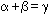EzMath supports variants for how to say things like powers. The following are all equivalent:

    x to the power of 3
x cubed
x^3


as are:

    x to the power of 2
x squared
x^2


Identifiers consist of a single letter optionally followed by one or more digits. The "times" operator is often implicit, as below for bx, which is interpreted as b times x. For instance, the following are equivalent:

    a times x squared plus b times x plus c equals 0
ax^2+bx+c=0


which yields:The implicit times operator is useful for expressions such as:

    (x+1)(x-1) = x(x-1) + (x-1) = x^2 - 1


In x(x-1) and f(x-1), which is multiplication and which is function application? EzMath requires you to make this explicit using the "function" keyword, as in:

   function f(x) = ax+b


which simply yields: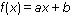If you are going to use a function identifier several times it is convenient to declare it as a function in advance:

    declare f as function; y = f(a) + f(b)


The keyword "wrt" is used as an abbreviation for "with respect to" for integrals and derivatives, as in:

    integral from 0 to pi of sin ax wrt x


which yields: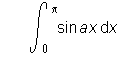The keyword "wrt" avoids the ambiguities that "d" would introduce. Would the "d" the identifier 'd' or does it introduce the variable of integration? Here are some examples for derivatives:

    derivative of f wrt x
2nd derivative of f wrt x
partial derivative of f wrt x
total derivative of f wrt x


Ezmath supports 1st, 2nd, 3rd, 4th, 5th etc. and "n th". For the latter a space is needed between the letter 'n' and the "th" since "nth" would be misinterpreted as the sequence of identifiers 'n', 't' and 'h'. Limits are represented as follows:

    limit as x tends to infinity of function f(x)


which yield:Intervals are represented as:

    open interval from a to b
closed interval from a to b
open closed interval from a to b
closed open interval from a to b


EzMath supports vectors, matrices and determinants, for instance:

    vector V
vector (3, 2, 7)
unit vector j

matrix M
matrix (1, 2; 2, 1)

inverse of matrix M
transpose of matrix M

determinant (1, 2; 2, 1)


where comma is used to separate items on the same row and semicolon to separate items on separate rows. Identifiers for vectors and matrices are rendered in a bold upright font. For example, typing:

    matrix M = matrix (1,2;2,1)


yields: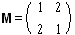and

    determinant (a sub 11, a sub 12; a sub 21, a sub 22)
= a sub 11 a sub 22 - a sub 21 a sub 12


yields: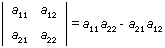The following illustrates set operations in EzMath:

    set S
set of x such that y

a union b

x is in S
x is a member of S

x is not in S
x is not a member of S


Support for Vector Calculus is still at a tentative stage:

    div vector E = 4 pi rho
curl vector B = 0


Currently div and curl are rendered as "div" and "curl" respectively. To show these functions with the nabla symbol you need to write:

    nabla dot vector E = 4 pi rho
nabla cross vector B = 0


EzMath includes some presentation features, e.g.

    bold x              renders x in bold font
cursive S           renders S in a cursive font
italic I            renders I in an italic font
upright F           renders F in an upright font

bigger X            renders X bigger than normal
smaller X           renders X smaller than normal

x bar               places a bar above the x
x hat               places a 'hat' above the x

x sub c             subscript
x sup alpha         superscript


You can define things for later use, effecting how something is rendered and what it means:

    declare U, V, W as vector; U cross V


thereafter in the current scope any occurrence of the identifiers U, V or W are interpreted as vectors. This information is retained when converting to MathML, see section 3.3.

Somewhat more speculative is the form:

    for any F let F bar denote inverse F


which defines a mapping from a presentation form to its semantics. This is used when translating to MathML, see section 3.3

### 3.2 Using EzMath in HTML Pages

A free plugin for EzMath is available from HP Labs. At the time this paper was written (November '97), we had developed a Netscape plugin for use on Windows 95/NT. We hope to port this to the Macintosh and to provide an ATL based ActiveX control for Windows by the time of the WWW'7 conference.

The Netscape plugin for use with Navigator 3.0 or 4.0 is invoked using the EMBED element, e.g.

    <EMBED type="text/ezmath" width="100%" height="70"
math="x = {-b plus or minus square root of {b^2 - 4ac}}/2a">


The width and height parameters are required. It would much easier if these could be supplied by the plugin itself, based upon the rendered size of the expression, but unfortunately Netscape Navigator doesn't allow this.

If you are using Netscape 4.0 or above, you can use the OBJECT element, as in:

    <OBJECT type="text/ezmath" width="100%" height="70">
<PARAM name=math
value"x = {-b plus or minus square root of {b^2 - 4ac}}/2a">
x = {-b plus or minus square root of {b^2 - 4ac}}/2a
</OBJECT>


This representation is compatible with HTML 4.0 and will also work with Internet Explorer using the ActiveX control for EzMath. Once again width and height are both required. We are told that for IE 4.0, it should be practical to use the simpler form:

    <OBJECT type="text/ezmath">
x = {-b plus or minus square root of {b^2 - 4ac}}/2a
</OBJECT>


but were unable to get sufficient information from Microsoft by the time of writing to test this.

If you want to include an expression as part of a text line, you may find align=absmiddle helps. It is unfortunate that neither Netscape nor Microsoft yet support a robust means to vertically align a plugin/control so that the math baseline is aligned with the baseline of the surrounding text.

When you click with the right or left mouse buttons on expressions rendered with the EzMath control, a dialog box appears. This offers the following features:

• The ability to change the font size
• The ability to change the expression or to pick from a menu of built-in examples
• The ability to copy to the clipboard the markup for the current expression (this sets the width and height parameters to match the expression).
• The ability to copy to the clipboard the MathML markup corresponding to the current expression.
• A link to further information on EzMath.

A standalone editor for EzMath is also available for Windows 95/NT. The majority of the EzMath code is written in ANSI C and is platform independent. A platform dependent part is used to interface to the platform and plugin specific code.

The platform independent part consists of the following:

• Dictionary of operators and reserved terms
• Lexical analyser mapping the input to a token sequence
• Operator precedence parser for expressions
• Function parsers specific to each kind of function
• Layout rules that map expressions to nested boxes
• Rendering rules for different kinds of boxes
• Rules for mapping expressions to MathML

### 3.3. EzMath and MathML

The W3C working group for Mathematics has developed an XML based representation for mathematical expressions. MathML is not suited for hand entry and has been designed for program to program interchange of mathematical expressions. It is not concise, but it is straight forward to manipulate and to render.

The MathML tag set has two parts: one part designed for capturing the visual presentation of mathematical notations and the other which is designed to describe the meanings of a small but widely used subset of mathematics (Kindergarten though end of high school). This part is generally referred to as the MathML content tag set.

The EzMath plugin and standalone editor can be used as a convenient way to create MathML content tags. The following example contrasts the EzMath and corresponding MathML: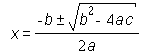EzMath:  x = {-b plus or minus square root of {b squared - 4ac}}/2a

MathML:
<e>
<eq/>
<ci>x</ci>
<apply>
<over/>
<apply>
<fn>
<mo>&PlusMinus;</mo>
</fn>
<apply>
<minus/>
<ci>b</ci>
</apply>
<apply>
<root/>
<apply>
<minus/>
<apply>
<power/>
<ci>b</ci>
<cn>2</cn>
</apply>
<apply>
<times/>
<apply>
<times/>
<cn>4</cn>
<ci>a</ci>
</apply>
<ci>c</ci>
</apply>
</apply>
<cn>2</cn>
</apply>
</apply>
<apply>
<times/>
<cn>2</cn>
<ci>a</ci>
</apply>
</apply>
</e>


MathML content tags use a prefix notation where the operator appears first followed by its operands. The APPLY element is used as a container for the operator and its operands. The E element is used in place of APPLY for relational operators such as equals, which is represented as <EQ/>. Entities are used for special symbols such as &plusmn; which denotes the symbol for "plus or minus".

### 3.4. Comparision of EzMath and Eqn

Because of the similarities between EzMath and eqn, it is interesting to make a closer comparison. Eqn was developed for use as part of the troff typesetting tool provided with Unix.

Eqn is more fussy than EzMath about the use of white space. For instance Greek letters aren't recognized unless delimited by space, thus f( theta ) is ok, but not f(theta).

When you want to use both subscripts and superscripts, e.g. to expressEzMath treats the following as equivalent:

    a sub k sup 2
a sup 2 sub k


Eqn is sensitive to the order in which sub and sup occur. If the sup comes before the sup the subscript is applied to the superscript and not to the base! This approach was rejected for EzMath based upon early feedback from would be users.

Eqn is much more limited than EzMath when it comes to being explicit about the meaning. For instance, eqn doesn't provide special syntax for derivatives:

  Ezmath:       derivative of y wrt x
Eqn:          dy / dx


As previously noted this makes it harder to provide effective renderings to speech or to export the meaning to mathematical manipulation packages.

To define matrices in eqn, you define columns of items using the above keyword to separate items. Each column is preceded by lcol, ccol or rcol, which indicate whether the items in the column should be left, center or right aligned within the column:

    matrix {
lcol 1 above 3
rcol 2 above 4
}


EzMath takes a different approach, using comma to separate items in the same row, and semicolon to separate rows:

    matrix (1, 2; 3, 4)


## 4. Formal definition of EzMath

EzMath is basically an English language transliteration of the MathML content tags. This makes it easier to understand, easier to write, and in most cases considerably more concise than MathML.

### 4.1. EzMath Grammar

In the following grammar non-terminals are in upper case. Square brackets are used to group things. The ? * + and & characters are used as follows:

FOO?
foo can occur zero or one times in this position
FOO*
foo can occur zero or more times in this position
FOO+
foo can occur one or more times in this position
[FOO BAR]&
foo and bar must occur once but may do so in any order
[FOO? BAR?]&
foo and bar can occur once only but may do so in any order
STATEMENT
::=     EXPRESSION [LIST-DELIM EXPRESSION]* ["TEXT"]? [.]

EXPRESSION

::=     BRACKETED-EXPRESSION
::=     FUNCTION
::=     PREFIX-OP [of]? EXPRESSION
::=     EXPRESSION INFIX-OP EXPRESSION
::=     EXPRESSION ORDINAL-OP EXPRESSION
::=     EXPRESSION POSTFIX-OP
::=     NUMBER
::=     IDENTIFIER

BRACKETED-EXPRESSION

::=     LEFT-BRACKET EXPRESSION?
[LIST-DELIM EXPRESSION]* RIGHT-BRACKET

LEFT-BRACKET
::=     {
::=     (

RIGHT-BRACKET
::=     }
::=     )

LIST-DELIM
::=     ,
::=     ;

FUNCTION

::=     CORE-FUNC [of]? EXPRESSION
::=     FUNCTION
::=     INTEGRAL
::=     SET
::=     MATRIX
::=     VECTOR
::=     COMPLEX
::=     DERIVATIVE
::=     LIMIT
::=     SUM
::=     PRODUCT
::=     DETERMINANT
::=     EITHER
::=     INTERVAL
::=     EQUATION
::=     LOG
::=     ROOT
::=     DECLARE
::=     FOR

CORE-FUNC

::=     abs
::=     mod
::=     sin
::=     cos
::=     tan
::=     sec
::=     cotan
::=     sinh
::=     cosh
::=     tanh
::=     sech
::=     csch
::=     coth
::=     arcsin
::=     arccos
::=     arctan
::=     exp
::=     ln
::=     div
::=     curl

FUNCTION
::=     function FN-NAME OF?
::=     function FN-NAME ( EXPRESSION )

FN-NAME
::=     EXPRESSION

OF
::=     of EXPRESSION

INTEGRAL
::=     integral [FROM? TO? OF? ]& WRT?
::=     integral over DOMAIN OF? WRT?

DOMAIN
::=     EXPRESSION

FROM
::=     from EXPRESSION

TO
::=     to EXPRESSION

WRT
::=     wrt EXPRESSION

LIMIT
::=     limit AS-TENDS-TO? OF?

AS-TENDS-TO
::=     as EXPRESSION tends to EXPRESSION

DERIVATIVE
::=     derivative OF? WRT?

MATRIX
::=     matrix BRACKETED-EXPRESSION
::=	    matrix IDENTIFIER

VECTOR
::=     vector BRACKETED-EXPRESSION
::=     vector IDENTIFIER

COMPLEX
::=     complex (real , imaginary)
::=     complex IDENTIFIER

INTERVAL
::=	    [open? closed?]& interval [FROM? TO?]&

SUM
::=     sum [FROM? TO? OF?]&

PRODUCT
::=     product [FROM? TO? OF?]&

DETERMINANT
::=     determinant BRACKETED-EXPRESSION
::=     determinant IDENTIFIER

EITHER
::=     either EXPRESSION WHEN? [or EXPRESSION WHEN?]*

WHEN
::=     when EXPRESSION

EQUATION
::=     equation EXPRESSION [:] EXPRESSION

LOG
::=     log of? EXPRESSION base EXPRESSION?

ROOT
::=     square root of? EXPRESSION
::=     EXPRESSION ORDINAL-OP root of? EXPRESSION

DECLARE
::=     declare IDENTIFIER [, IDENTIFIER] as TYPE

FOR
::=     for any IDENTIFIER [, IDENTIFIER]
let EXPRESSION denote EXPRESSION


### 4.2. Lexical Syntax

The following describes the rules used by the EzMath tokenizer:

#define IsWhite(c) (c == ' ' || c == '\t' || c == '\n' || c== '\r')
#define IsLetter(c) (('a' <= c && c <= 'z') || ('A' <= c && c <= 'Z'))
#define IsDigit(c) ('0' <= c && c <= '9')
#define IsAlphaNumeric(c) (IsLetter(c) || IsDigit(c))
#define IsVisible(c) (32 < c && c < 127)
#define IsSymbol(c) (!IsLetter(c) && !IsDigit(c) && IsVisible(c))


Symbol tokens consist of one or more characters matching the IsSymbol set. These are generally used for operators or punctuation.

Name tokens start with a letter matching IsLetter followed by characters matching IsLetter or IsDigit. EzMath's tokeniser recognizes terms defined in the dictionary consisting of several name or symbol tokens separated by white space, e.g.

    x to the power of y

is tokenized as the 3 tokens:

   "x", "to the power of" and "y"

while "sin ax" is tokenized as "sin", "a" and "x". Greek letters are denoted by reserved words e.g."alpha" for lower case alpha and "Alpha" for upper case alpha. In the case that several dictionary terms match the current lexical position, the longest known term is used. The dictionary classifies each term into the categories: number, identifier, operator or function. Each term may be associated with methods for parsing and formatting.

### 4.3. Table of Operators

Description Symbol/name Precedence Grouping Aliases Comments
prefix partial 800 right   derivatives
prefix total 800 right   derivatives
prefix unit vector 800 right   see "vector"
prefix transpose 800 right   matrix or vector
prefix inverse 800 right   matrix or function
prefix open 800 right   intervals
prefix closed 800 right   intervals
prefix double 800 right   integrals
prefix triple 800 right   integrals
prefix surface 800 right   integrals
prefix volume 800 right   integrals
prefix contour 800 right   integrals
infix superscript 800 right sup not for powers
infix subscript 800 right sub and  _
infix modulo 800 right mod
postfix factorial 780 left ! e.g. 6!
postfix per cent 775 left %
postfix degrees 770 left   angles
postfix minutes 770 left   angles
postfix seconds 770 left   angles
prefix variance 770 right var statistics
prefix median 770 right   statistics
prefix mode 770 right   statistics
prefix mean 770 right   statistics
prefix standard deviation 770 right sdev statistics
prefix moment 770 right   statistics
postfix prime 770 left   accent
postfix double prime 770 left   accent
postfix tilde 770 left   accent
postfix hat 770 left   accent
postfix bar 770 left   accent
postfix dot 770 left   accent
postfix double dot 770 left ddot accent
postfix arrow 770 left   accent
prefix bold 770 right   font style
prefix upright 770 right   font style
prefix italic 770 right italic font style
prefix cursive 770 right   font style
prefix smaller 770 right   font style
prefix bigger 770 right   font style
postfix st 770 left   ordinal
postfix nd 770 left   ordinal
postfix rd 770 left   ordinal
postfix th 770 left   ordinal
infix to the power of 760 right ^ power
postfix squared 760 left ^2 power
postfix cubed 760 left ^3 power
prefix plus 600 right +
prefix minus 600 right -
prefix not 600 right -
infix fraction 580 right   renders as x/y
infix divide 580 right   special divide sign
infix circle times 520 right
infix circle plus 520 right
infix circle minus 520 right
infix dot 500 right
infix cross 500 right
infix composed with 500 right   function composition
infix times 480 right *
infix over 450 right
infix / 450 right
infix plus 400 right +
infix minus 400 right -
infix plus or minus 400 right
infix intersection 380 right intersect sets
infix union 360 right   sets
infix is superset of 360 right   sets
infix is superset or equals 360 right   sets
infix is not a superset of 360 right   sets
infix is subset of 360 right   sets
infix is subset or equals 360 right   sets
infix is not a subset of 360 right   sets
infix is in 360 right is a member of set membership
infix is not in 360 right is not a member of set membership
infix maps to 340 right
infix equals 300 left = comparison
infix equivalent 300 left equiv comparison
infix congruent 300 left   comparison
infix less than 300 left < comparison
infix greater than 300 left > comparison
infix not equals 300 left != comparison
infix less than or equals 300 left <= comparison
infix greater than or equals 300 left >= comparison

 when tends to base denote from to wrt of if and or . : , ;

### 4.5. Tables of Symbols

Greek upper case letters
Alpha Beta Chi Delta
Epsilon Phi Gamma Eta
Iota Kappa Lambda Mu
Nu Omicron Pi Theta
Rho Sigma Tau Upsilon
Omega Xi Psi Zeta

Greek lower case letters
alpha beta chi celta
epsilon phi gamma eta
iota kappa lambda mu
nu omicron pi theta
rho sigma tau upsilon
omega xi psi zeta

Miscellaneous named symbols
for all there exists therefore proportional
angle perpendicular infinity nabla
omega pi curly phi curly Upsilon curly theta
double arrow left arrow right arrow up arrow
down arrow horizontal bar vertical bar bullet
ellipsis aleph imaginary real
weierstrass empty set bra ket
lfloor rfloor lceil rceil

## 5. References

 "UNIX Text Processing", Dale Dougherty and Tim O'Reilly, Hayden Books Indianapolis, 1987. ISBN 0-672-46291-5

 "TEX for the Impatient", Paul W. Abrahams, Addison Wesley Reading Mass., 1990. ISBN 0-201-51375-7

 "LATEX : A Documentation Preparation System, 2nd edition", Leslie Lamport, Addison Wesley Reading Mass., 1994. ISBN 0-201-52983-1

 "AMS TEX Resources", American Mathematical Society 1997, http://www.ams.org/TeX/home.html

 "ISO 12083 DTD", ISO 12083:1993, Information and Documentation -- Electronic Manuscript Preparation and Markup, Geneva, 1993.

 "AAP Math DTD", ANSI/NISO Z39.59-1988, Standard for Electronic Manuscript Preparation and MarkUp, Bethesda, 1988.

 "Practical SGML", Eric van Herwijnen, Kluwer Academic Publishers Norwell Mass., 1994. ISBN 0-7923-9434-8

 "Mathematica", Wolfram Research, Urbana Champagne Illinois, http://www.wolfram.com/mathematica/

 "Maple", Waterloo Maple, Ontario. http://www.maplesoft.on.ca/

 "HP Labs", Hewlett Packard Laboratories, Bristol England, http://www.hpl.hp.co.uk/

 "HTML 4.0", W3C Recommendation for HTML 4.0, December 1997, http://www.w3.org/TR. For an easy to read user guide see, "Raggett on HTML 4", Dave Raggett, Jenny Lam, Ian Alexander & Michael Kmiec, "Addison Wesley Longman Reading Mass., 1997. ISBN 0-201-17805-2

 "MathML", W3C Recommendation for the Mathematical Markup Language, January 1998, http://www.w3.org/TR

 "Standard dtd's and scientific publishing", N.A.F.M. Poppelier, E. van Herwijnen, C.A. Rowley, EPSIG News 5 (1992) #3, September 1992, p. 10-19.